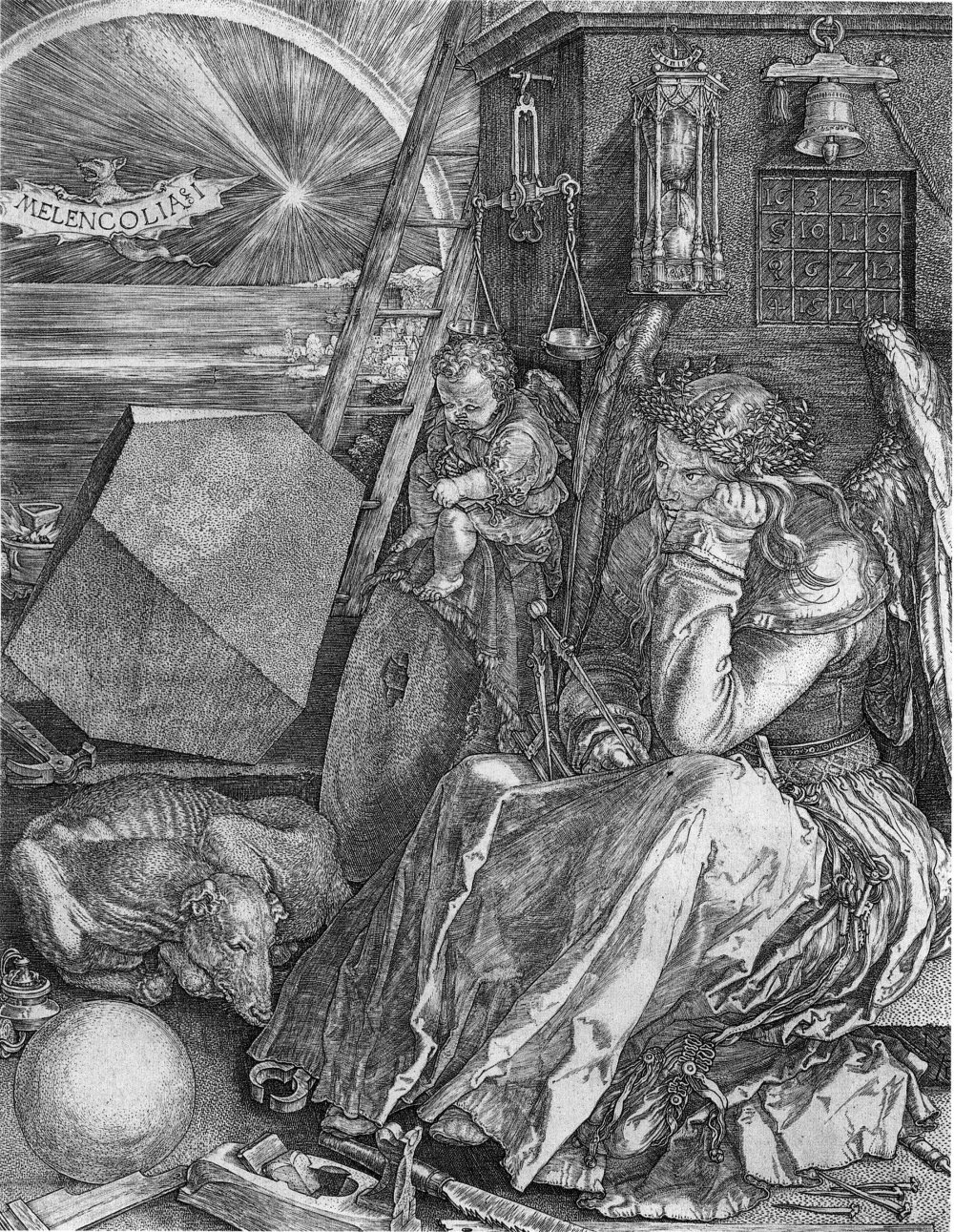M A T H 2 1 B
Mathematics Math21b Spring 2008
Linear Algebra and Differential Equations
Exhibit: latin squares
Office: SciCtr 434

## Latin squares

A nxn matrix is called a latin square if the numbers 1,...,n occur exactly once in each row and exactly once in each column of the matrix. A simple example is
``` A =   | 1   2 |
| 2   1 |
```
An example of a 3x3 latin square, you have encountered in the first midterm. Here is an example of a 5x5 latin square:
```A = | 1 2 3 4 5 |
| 2 3 5 1 4 |
| 3 5 4 2 1 |
| 4 1 2 5 3 |
| 5 4 1 3 2 |
```

## Suduku squares

 A 9x9 matrix is a Suduku square, if it is a latin square and if additionally in each of the 9 3x3 submatrices all numbers 1..,9 occur exactly once too. Here is an example: ```A = | 3 4 2 9 7 8 1 5 6 | | 6 9 5 2 4 1 3 7 8 | | 1 7 8 6 3 5 2 4 9 | | 7 6 3 4 9 2 5 8 1 | | 8 1 9 3 5 7 4 6 2 | | 2 5 4 8 1 6 7 9 3 | | 9 3 7 1 6 4 8 2 5 | | 4 8 1 5 2 9 6 3 7 | | 5 2 6 7 8 3 9 1 4 | ```

## Magic squares

 A nxn matrix is called a Magic square if it contains all integers 1,...,n2 exactly once and each row each column and each diagonal column has the property that the sum is constant. An example: ```| 4 9 2 | | 3 5 7 | | 8 1 6 | ``` Note that this square appears in the center of the above Suduku matrix which has been found by Paul Muljadi.The magic square which appears in Durers Melancolia I is ```A = | 16 3 2 13 | | 5 10 11 8 | | 9 6 7 12 | | 4 15 14 1 | ```